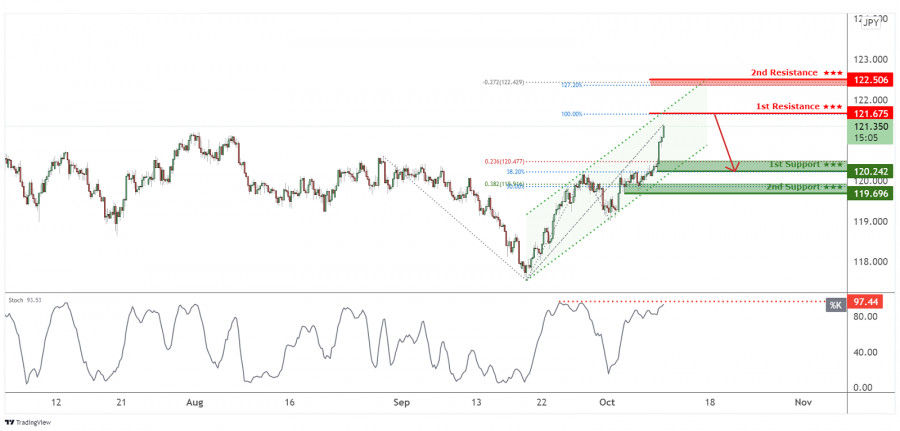# CHFJPY facing bearish pressure, drop incoming!

## CHFJPY is approaching the upper resistance of the ascending price channel in line with 1st resistance at 121.675 in line with Horizontal swing high and 100% Fibonacci extension . Price is likely to reverse off from 1st resistance at 121.675 in line with Horizontal swing high and 100% Fibonacci extension to take 1st support at 120.242 in line with 23.6% Fibonacci retracement and 78.6% Fibonacci extension level. Our bearish bias is further supported by how Stochastic is approaching resistance where price dropped in the past. Otherwise price may bullish towards 2nd resistance at 122.506 in line with -27.2% Fibonacci retracement and 127.2% Fibonacci extension.Trading RecommendationEntry: 121.675Reason for Entry:Horizontal swing high and 100% Fibonacci extensionTake Profit: 120.242Reason for Take Profit:23.6% Fibonacci retracement and 78.6% Fibonacci extension level Stop Loss: 122.506Reason for Stop Loss: -27.2% Fibonacci retracement and 127.2% Fibonacci extensionThe material has been provided by InstaForex Company - www.instaforex.comCHFJPY is approaching the upper resistance of the ascending price channel in line with 1st resistance at 121.675 in line with Horizontal swing high and 100% Fibonacci extension . Price is likely to reverse off from 1st resistance at 121.675 in line with Horizontal swing high and 100% Fibonacci extension to take 1st support at 120.242 in line with 23.6% Fibonacci retracement and 78.6% Fibonacci extension level. Our bearish bias is further supported by how Stochastic is approaching resistance where price dropped in the past. Otherwise price may bullish towards 2nd resistance at 122.506 in line with -27.2% Fibonacci retracement and 127.2% Fibonacci extension.

Entry: 121.675

Reason for Entry:

Horizontal swing high and 100% Fibonacci extension

Take Profit: 120.242

Reason for Take Profit:

23.6% Fibonacci retracement and 78.6% Fibonacci extension level

Stop Loss: 122.506

Reason for Stop Loss:

-27.2% Fibonacci retracement and 127.2% Fibonacci extension

The material has been provided by InstaForex Company - www.instaforex.com